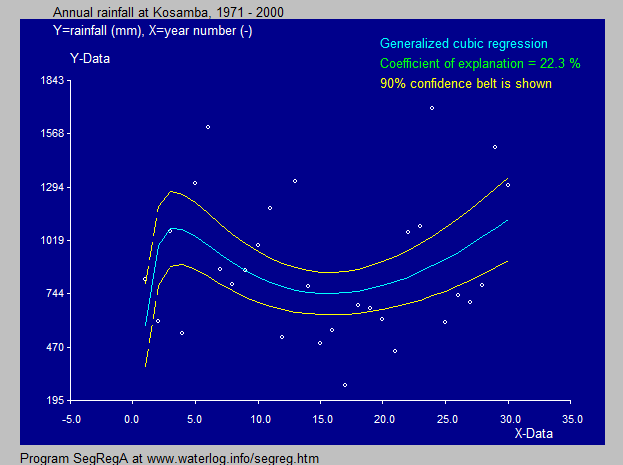# SegRegA :   segmented regression with breakpoint as well as generalized S-curve, Power curve, Quadratic and Cubic functions. Totally free download of software

 Summary: The SegRegA computer program (calculator) is designed to perform a segmented regression analysis, but in addition it offers the opportunity to apply S-curve, Power function, quadratic and third degree regressions. The last three have an increased versatility by applying an exponential transformation of the data by assuming a range of exponents and selecting the one that produces the best fit. Thus, the exponent is optimized. The cubic regression uses the technique of inversed matrices and determinants to obtain the least squares result. An example of the application of the generalized cubic regression can be found in the article: "Applying SegRegA to the annual average temperature trend from 1900 to 2020 in the Netherlands resulting from global warming: analysis by segmented linear regression types and curved functions such as the S-curve, Power curve, generalized quadratic and cubic regressions". Download from: average temperature.pdf Experiences: For improvement, I am interested to learn about your experiences with SegRegA. For this there is a contact form. Screenprint of SegRegA showing the regression options.SegRegA

Go to:

Software
& models

General articles
& manuals

Artiículos
(in Spanish,
en Español)

Published
reports & cases

Particular
reports & cases

FAQ'sThe equation in the potato example (above) is found by a transformation raising X to the power 0.5 so that W = X ^ 0.5 and then performing a cubic regression of Y on W with the result: Y = 0.537 W ^ 3 - 4.70 W ^ 2 + 11.2 W + 1.84 and hence: Y = 0.537 X ^ 1.5 - 4.70 X + 11.2 X ^ 0.5 + 1.84 which is now no longer a third degree (cubic) equation. See also this potato article